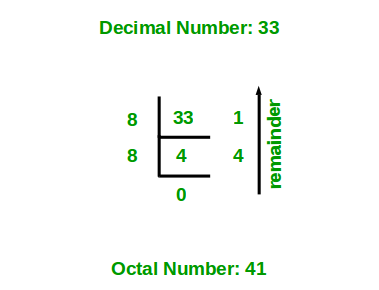# Java Program For Decimal to Octal Conversion

• Last Updated : 29 Apr, 2021

Given a decimal number N, convert N into an equivalent octal number i.e convert the number with base value 10 to base value 8. The decimal number system uses 10 digits 0-9 and the octal number system uses 8 digits from 0-7 to represent any numeric value.

Illustration:

```Input : 33
Output: 41

Input : 10
Output: 12```

Approach 1:

1. Store the remainder when the number is divided by 8 in an array.
2. Divide the number by 8 now
3. Repeat the above two steps until the number is not equal to 0.
4. Print the array in reverse order now.Example of converting the decimal number 33 to an equivalent octal number.

Example:

## Java

 `// Java program to convert a Decimal Number to Octal Number` `// Importing input output classes``import` `java.io.*;` `// Main class``class` `GFG {` `    ``// Method``    ``// To convert decimal to octal``    ``static` `void` `decToOctal(``int` `n)``    ``{``        ``// Creating an Integer array of``        ``// array to store octal number``        ``int``[] octalNum = ``new` `int``[``100``];` `        ``// counter for octal number array``        ``int` `i = ``0``;``        ``while` `(n != ``0``) {` `            ``// Storing remainder in octal array``            ``octalNum[i] = n % ``8``;``            ``n = n / ``8``;``            ``i++;``        ``}` `        ``// Printing octal number array in reverse order``        ``for` `(``int` `j = i - ``1``; j >= ``0``; j--)``            ``System.out.print(octalNum[j]);``    ``}` `    ``// Method 2``    ``// Main driver method``    ``public` `static` `void` `main(String[] args)``    ``{``        ``// Custom input Integer number``        ``int` `n = ``33``;` `        ``// Calling the above method to convert``        ``// Decimal to Octal number``        ``decToOctal(n);``    ``}``}`

Output
`41`

Time Complexity: O(log N)

Approach 2:

• Initialize ocatalNum with 0 and countVal with 1 and the decimal number as n
• Calculate the remainder when decimal number divided by 8
• Update octal number with octalNum + (remainder * countval)
• Increment the countval by countval*10
• Divide the decimal number by 8
• Repeat from step 2 until the decimal number is zero

Example:

## Java

 `// Java Program to Convert Decimal Number to Octal Number` `// Importing input output classes``import` `java.io.*;` `// Main class``class` `GFG {` `    ``// Method 1``    ``// To calculate the octal value of the given``    ``// decimal number``    ``static` `void` `decimaltooctal(``int` `deciNum)``    ``{` `        ``// Initially declaring and initializing the``        ``// octal number with zero``        ``int` `octalNum = ``0``, countval = ``1``;``        ``int` `dNo = deciNum;` `        ``// Condition check``        ``while` `(deciNum != ``0``) {` `            ``// Decimals remainder is calculated``            ``int` `remainder = deciNum % ``8``;` `            ``// Storing the octalvalue``            ``octalNum += remainder * countval;` `            ``// Storing exponential value``            ``countval = countval * ``10``;``            ``deciNum /= ``8``;``        ``}` `        ``// Print and display the octal number``        ``System.out.println(octalNum);``    ``}` `    ``// Method 2``    ``// Main driver method``    ``public` `static` `void` `main(String[] args)``    ``{``        ``// Custom input decimal number``        ``int` `n = ``33``;` `        ``// Calling the Method1 to convert above number``        ``// to Octal number system``        ``decimaltooctal(n);``    ``}``}`

Output
`41`

Time Complexity: O(log N)
Auxiliary Space: O(1)

My Personal Notes arrow_drop_up